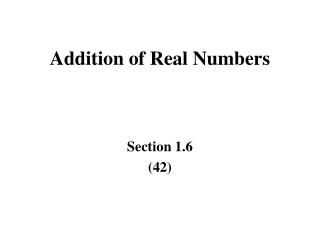DownloadDownload PresentationTélécharger la présentation- - - - - - - - - - - - - - - - - - - - - - - - - - - E N D - - - - - - - - - - - - - - - - - - - - - - - - - - -
##### Presentation Transcript

1. Addition of Real Numbers Section 1.6 (42)

2. Objectives(41) Add real numbers using the number line Add fractions Identify opposites (additive inverses) Add using absolute values Note: We don’t cover Using Calculators

3. 1.6.1 Add Real Numbers Using a Number Line(41) We first locate the first point. If we add a positive number, we move over that number of units to the right. If we add a negative number, we move over that number of units to the left. Example: -2 + ( +3 ) -4 -3 -2 -1 0 1 2 3 4 o - - - 3 - -x 1

4. Examples +3 + (-5) -4 -3 -2 -1 0 1 2 3 4 o x - - - - 5 - - - - - -2 -2 + (+2) -4 -3 -2 -1 0 1 2 3 4 o - - - - x 0

5. Example A miner descents 120 feet down a mine shaft. Later, he descends another 145 feet. Find the depth of the descent. Given: Initial 120 feet down (-120) Later 145 feet further down (-145) Find: Total distance down How: Add initial and distance down Solve: -120 + ( -145 )

6. Only a mad man would try and do this using a vertical number line. If we add two negative numbers, we add the absolute values and put a minus in front. -120 + -145 -265 The miner would have descended 265 feet (-265)

7. 1.6.2 Add Fractions When you add fractions, you MUST have the same denominators. You have to find the LCD and create equivalent fractions with that denominator. Then just add the numerators. Example: 1/2 + 2/3 LCD = 6 1/2 (3/3) + 2/3 (2/2) 3/6 + 4/6 = (3 + 4)/6 = 7/6 = 1 1/6

8. Examples 5/8 + (-6/7) LCD 56 5/8 (7/7) + (-6/7) (8/8) 35/56 + (-48/56) [ +35 + (-48) ] / 56 -13/56 1/8 + ( -7/12 ) LCD 24 1/8 (3/3) + (-7/12) (2/2) 3/24 + (-14/24) [ +3 + (-14)] / 24 -11/24

9. 1.6.3 Identify Opposites(44) The purpose for opposites is to determine which value to add to make the sum equal 0. They are better known as additive inverses. The additive inverse is the same value with the opposite sign. Examples: the opposite of 3.5 is -3.5 the opposite of -3/4 is +3/4 the opposite of 0 is 0

10. 1.6.4 Add Using Absolute Values(44) Same sign: To add two numbers with the same sign (either both positive or both negative), add their absolute values and use the same sign. Examples: ( +10 ) + ( +5 ) = +15 ( -9 ) + ( +5 ) = -4

11. Using Absolute Values (cont)(44) Different signs. To add two numbers with different signs (one positive, the other negative), subtract the absolute value of the smaller from the absolute value of the larger and use the sign of the larger. Examples: ( -9 ) + ( +4 ) = -5 ( -2 ) + ( +8 ) = +6

12. Examples -1/4 + ( +3/4) -2/4 = -1/2 5/6 + ( -1/9 ) LCD 18 5/6 ( 3/3) + ( -1/9 ) ( 2/2) 15/18 + ( -2/18 ) = +13/18 -2/5 + ( +1/4 ) LCD 20 -2/5 ( 4/4) + ( +1/4 ) ( 5/5 ) -8/20 + ( + 5/20 ) = -3/20

13. Example A bakery had a profit of \$450,567 for the first five months of the year, and a loss of \$52,987 for the remainder of the year. Find the net-profit or loss for the year. Given: \$450,567 profit Jan – May \$52,987 loss Jun – Dec Find: Net-profit or loss for the year How: Add the values from each set Solve: 450,567 + ( -52,987 )

14. 450,567 Since they are different signs, -52,987 subtract absolute values and 397,580 use the sign of the larger Solution: There was a net-profit of \$397,580 for the year.

15. Objectives(41) Add real numbers using the number line Add fractions Identify opposites (additive inverses) Add using absolute values Note: We don’t cover Using Calculators

16. Addition of Real Numbers Section 1.6 (42)